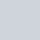Latest MCQs
Home > MCQs > Math > The ages of X and Y are in the ratio 7:4. If the sum of their present ages is 55 then the age of X is:

# The ages of X and Y are in the ratio 7:4. If the sum of their present ages is 55 then the age of X is:

A.20
B.25
C.30
D.35